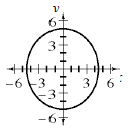### Home > A2C > Chapter 12 > Lesson 12.1.2 > Problem12-41

12-41.

Graph each ellipse.

1. $5x^{2} + 4y^{2} = 100$

Divide both sides by 100 to get the equation into graphing form.1. $\frac { ( x - 2 ) ^ { 2 } } { 25 } + \frac { ( y + 3 ) ^ { 2 } } { 9 } = 1$

The center is at $\left(2, −3\right)$.